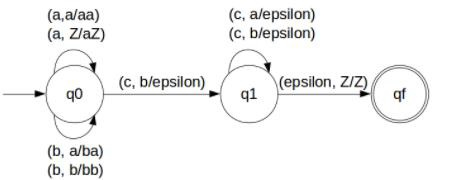# Construct DPDA for anbmc(n+m) n,m‚â•1 in TOC

A push down automata (PDA) can be formally described as seven tuples

(Q, Σ,S, δ,q0,I,F)

Where,

• Q is finite number of states
• Σ is input alphabet
• S is stack symbol
• Δ is the transition function: QX(ΣU{e})XSXQ
• q0 is the initial state (q0 belongs to Q)
• I is the initial state top symbol
• F is a set of accepting states (F belongs to Q)

## Problem

Construct PDA for anbmc(n+m) n,m≥1

## Solution

So, the strings which are generated by the given language are as follows−

L={abcc,aabccc,aaabbccccc,….}

That is to add the number of a's and b's, and that will equal the number of c's.

So for every a's and b's we will pop c's from the stack. Let’s count the number of a's and b's that total number is equal to the number of c's. It can be achieved by pushing a's and b's in STACK and then pop a's and b's whenever "c" comes.

Finally, at the end of the strings if nothing is left in the STACK then we can say that language is accepted in the PDA.

The PDA for the given problem is as follows −## Transition Functions

The transition functions are given below −

Step 1: δ(q0, a, Z) = (q0, aZ)

Step 2: δ(q0, a, a) = (q0, aa)

Step 3: δ(q0, b, a) = (q0, ba)

Step 4: δ(q0, b, b) = (q0, bb)

Step 5: δ(q0, c, b) = (q1, ε)

Step 6: δ(q1, c, b) = (q1, ε)

Step 7: δ(q1, c, a) = (q1, ε)

Step 8: δ(q1, ε, Z) = (qf, Z)

## Explanation

Step 1 − Let’s take input string: "aabbcccc" which satisfies the given condition.

Step 2 − Scan string from left to right.

Step 3 − For input 'a' and STACK alphabet Z, then,

Push the input 'a' into STACK: (a,Z/aZ) and state will be q0

Step 4 − For input 'a' and STACK alphabet 'a', then,

Push the input 'a' into STACK: (a,a/aa) and state will be q0

Step 5 − For input 'b' and STACK alphabet 'a', then,

Push the input 'b' into STACK: (b,a/ba) and state will be q0

Step 6 − For input 'b' and STACK alphabet 'b', then

Push the input 'b' into STACK: (b,b/bb) and state will be q0

Step 7 − For input 'c' and STACK alphabet 'b' and state is q0, then

Pop one 'b' as: (c,b/ε) and state will be q1

Step 8 − For input 'c' and STACK alphabet 'b' and state is q1, then

Pop one 'b': (c,b/ε) and state remain q1

Step 9 − For input 'c' and STACK alphabet 'a' and state is q1, then

Pop one 'a': (c,a/ε) and state will be q1

Step 10 − For input 'c' and STACK alphabet 'a' and state is q1, then

Pop one 'a' as: (c,a/ε) and state will be remain q1

Step 11 − We reached end of the string, for input ε and STACK alphabet Z, then

Go to final state(qf) : (ε, Z/Z)